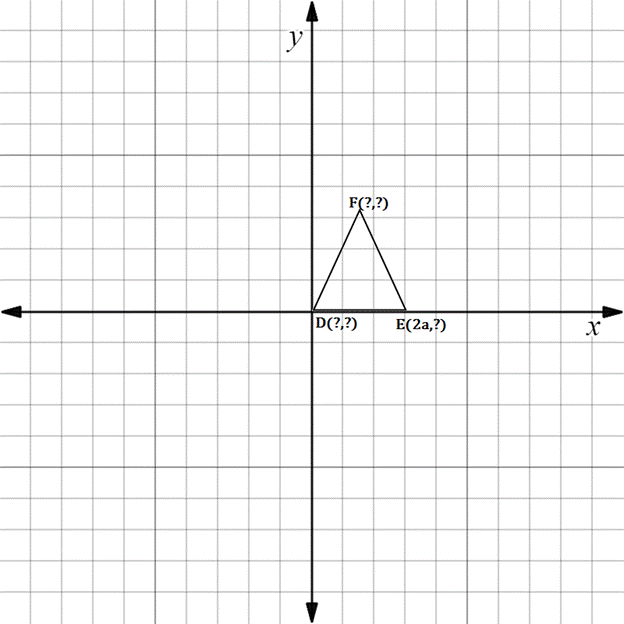Chapter 10.3, Problem 12EElementary Geometry For College St...

7th Edition
Alexander + 2 others
ISBN: 9781337614085

Solutions

Chapter
SectionElementary Geometry For College St...

7th Edition
Alexander + 2 others
ISBN: 9781337614085
Textbook Problem

In Exercises 11 to 16, supply the missing coordinates for the vertices, using as few variables as possible.D E F is an isosceles triangle with D F - = F E -

To determine

To find:

The missing coordinates for the vertices.

Explanation

The given figure is shown below.

From the figure, the following observations are made.

Since, the vertex D is at the origin.

The coordinates of D0, 0.

Now, consider the vertex E.

Here, x-coordinate is given as 2a.

In the E vertex, observe where it lies on y-axis.

Since, it is at 0, the vertex E has the coordinates 2a, 0.

The distance from D to E in the x-axis is 2a.

F lies midway between D and E

Still sussing out bartleby?

Check out a sample textbook solution.

See a sample solution

The Solution to Your Study Problems

Bartleby provides explanations to thousands of textbook problems written by our experts, many with advanced degrees!

Get Started

29. If, find the following. (a) (b) (c) (d)

Mathematical Applications for the Management, Life, and Social Sciences

Finding a Limit In Exercises 47-62, find the limit. limx0(x+x)2x2x

Calculus: Early Transcendental Functions (MindTap Course List)

In Exercises 110, find the graphical solution to each inequality. 3y+50

Finite Mathematics for the Managerial, Life, and Social Sciences